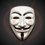# DOUBT in titrations!

An aqueous solution containing 0.10 g KIO3 ( formula weight =214.0) was treated with an excess of KI solution. The solution was acidified with HCl . The liberated I2 consumed 45 ml of thiosulphate solution to decolorize the blue starch iodine complex. Calculate the molarity of Na2S2O3 ??

My solution is
Redox change IO3- ---> I2 2IO3- + 12H+ + 10e- = I2 + 6H2O n factor of KIO3 = 5 Eq wt of KIO3 = 214/5 Gm eq of KIO3 = 0.10/(214/5) = Gm eq of I2 Let the molarity of Na2S2O3 = x N factor of Na2S2O3 = 1 (0.10 x 5)/(214) = (45/1000) x (x) x 1 or x = 0.052M But the solution is 0.062M What is wrong in my solution.Note by Rudraksh Singh
3 years ago

This discussion board is a place to discuss our Daily Challenges and the math and science related to those challenges. Explanations are more than just a solution — they should explain the steps and thinking strategies that you used to obtain the solution. Comments should further the discussion of math and science.

When posting on Brilliant:

• Use the emojis to react to an explanation, whether you're congratulating a job well done , or just really confused .
• Ask specific questions about the challenge or the steps in somebody's explanation. Well-posed questions can add a lot to the discussion, but posting "I don't understand!" doesn't help anyone.
• Try to contribute something new to the discussion, whether it is an extension, generalization or other idea related to the challenge.

MarkdownAppears as
*italics* or _italics_ italics
**bold** or __bold__ bold
- bulleted- list
• bulleted
• list
1. numbered2. list
1. numbered
2. list
Note: you must add a full line of space before and after lists for them to show up correctly
paragraph 1paragraph 2

paragraph 1

paragraph 2

[example link](https://brilliant.org)example link
> This is a quote
This is a quote
    # I indented these lines
# 4 spaces, and now they show
# up as a code block.

print "hello world"
# I indented these lines
# 4 spaces, and now they show
# up as a code block.

print "hello world"
MathAppears as
Remember to wrap math in $$ ... $$ or $ ... $ to ensure proper formatting.
2 \times 3 $2 \times 3$
2^{34} $2^{34}$
a_{i-1} $a_{i-1}$
\frac{2}{3} $\frac{2}{3}$
\sqrt{2} $\sqrt{2}$
\sum_{i=1}^3 $\sum_{i=1}^3$
\sin \theta $\sin \theta$
\boxed{123} $\boxed{123}$

Sort by:

have u tried it ??

- 3 years ago

http://exxamm.com/QuestionBank/practice/1119367210 THIS is the link bro ,, but sorry it is not the full length paper

- 3 years ago

Hey this is iit 1998 problem ... Thanks for responding bro !!

- 3 years ago

- 3 years ago

Thanks for tagging me. Let me try, then i will see ur solution, Then if it is different than urs, i will post it here.

- 3 years ago

- 3 years ago

@Prakhar Bindal , @Aniket Sanghi , @Shubham Dhull , @Harsh Shrivastava , @A E please guys help me out here ,,,

- 3 years ago